Artificial Intelligence & Software

# The Diffusion Model from a Patent Perspective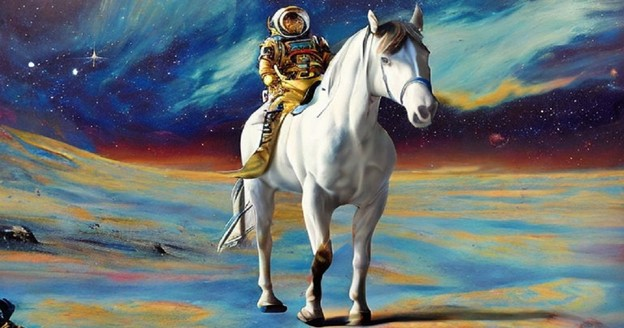Summary

A column analyzing filed patents related to diffusion models and providing insights into the trends and future outlook for the rightsizing of technology related to diffusion models.

With the advent of generative models such as Midjourney, DALL-E 2, and Stable Diffusion, anyone can easily create convincing images using artificial intelligence. At the same time, controversies and concerns related to generative models have exploded. In this article, we will analyze the patents filed for "diffusion models," one of the most recently researched generative models, and discuss the trends and prospects of patenting technologies related to diffusion models.

## Diffusion Model

A diffusion model is a type of generative model, which is an artificial intelligence model that generates data that didn't exist before but is similar to the training data.

A diffusion model progressively removes noise from the original data until it finally produces the data we want, which is the original data without any noise. This process of gradually removing noise from a diffusion model is called the denoising process, but how can a diffusion model perform denoising?

To train a diffusion model, we take one original image and add a predetermined amount of noise to it in small increments to create multiple noisy images. For example, consider a situation where we take an original image (X0) and add small bits of noise to it, so that after 1000 noises (T=1000), the original image is completely noisy (XT).

If we show the diffusion model the process of adding noise in small increments and then have it learn the opposite process of adding noise, i.e., subtracting noise in small increments, the trained model can take the noise as input and produce a slightly denoised image. The denoised image can then be fed back into the model to produce a denoised image twice, and so on, until eventually we have a complete, noise-free image.

Specifically, we'll create 1000 training images from one original image and give the diffusion model information about the number of times we added noise (t) and the resulting image after adding noise (Xt) as input of the model. By doing this, we can train the diffusion model to learn the conditional probability distribution of subtracting noise (pθ), which is the reverse of the process of adding noise, from the conditional probability distribution of adding noise(q).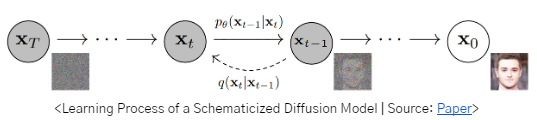By applying the basic structure of the diffusion model above, you can design models that embed additional text into the denoising process, or denoise a feature map rather than the image itself, and then decode it back to the original image.

Thanks to papers like <Denoising diffusion probabilistic models (DDPM)>, published in June 2020, and <Diffusion Models Beat GANs on Image Synthesis>, published in May 2021, diffusion models have emerged as a new stream of generative models and are now one of the most active research topics in the field of generative models. Recently, there has been an increasing number of attempts to utilize diffusion models to solve problems not only in images, but also in video and speech.

With all this interest in diffusion models, let's take a closer look at how many patents have been issued and how well they are protected.

## Patent applications for diffusion models by year

Learning about patent filing trends related to diffusion models, we analyzed data from K-wert, an artificial intelligence-based patent analysis database by using a proprietary data search formula. After removing noise from the approximately 1,500 patents detected using the search formula, a total of 76 patents were determined to be related to diffusion models.  Given that diffusion models are probably the most actively researched topic in the field of generative models, some readers may be surprised by the low number of patents related to them.

The idea of diffusion models was first presented in the 2015 paper <Deep Unsupervised Learning using Nonequilibrium Thermodynamics>, but it wasn't until 2021, as mentioned above, that diffusion models as a generative model gained traction. Given that patents are typically published one and a half years after they are filed, it appears that a large number of patents have been filed on diffusion models, many of which are still unpublished. We have also filed a patent utilizing diffusion models, but that patent is also currently unpublished.

Looking at the filing dates of the currently published patents, only 6 of the 76 patents were filed before 2021, but 6 were filed in 2021, 35 were filed in 2022, and 29 were filed between January and June in 2023. If we consider the number of patents filed but not published, the number of patents related to diffusion models seems to have exploded in 2021. Considering that 2020 and 2021 saw the publication of significant patents related to the aforementioned diffusion models, we can conclude that there is some alignment between patent filings and the latest research trends.

Looking at the filing trends by country, the majority of the 76 patents that have been published to date were filed in China: 68 in China, 6 in the U.S., and 1 in South Korea. The short period of time between the filing and publication dates of the patents filed in China suggests that many applicants in China are adopting a strategy of early disclosure of their technology after filing a patent application.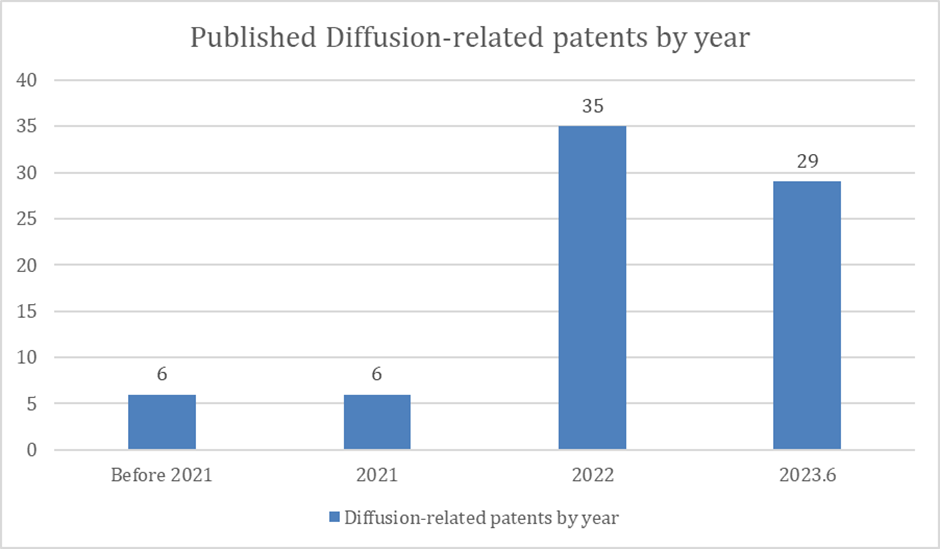<Patent applications for diffusion models by year>

## Patent applications for diffusion models based on data domain

Next, let's look at trends in patents related to diffusion models by data domain.

Here's a breakdown of the patent applications for diffusion model-related patents that have been published so far, by data domain.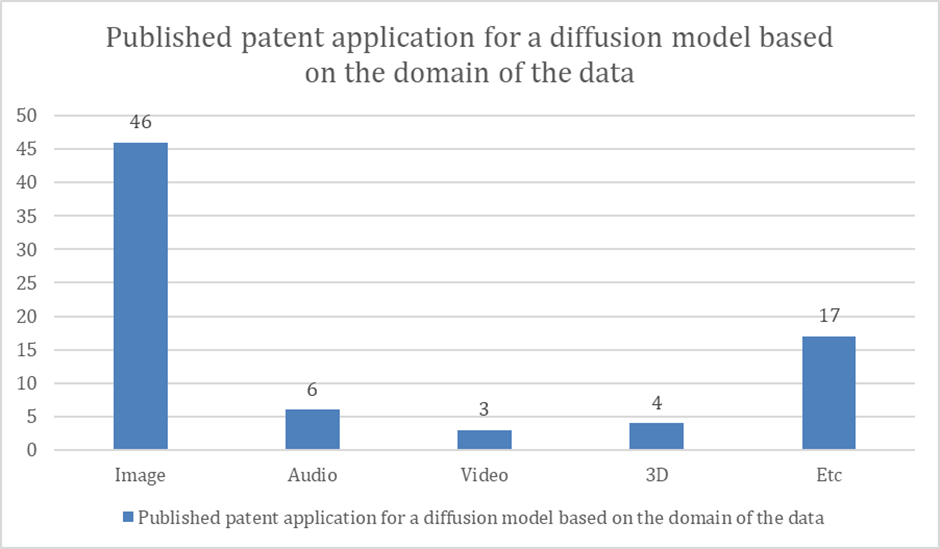< Published patent applications for diffusion models by the domain of the data>

From the chart, we can see that there are 46 patents for the image domain, compared to 3 and 6 published applications for the video and audio domains, respectively, and 4 published applications for the 3D domain. We can see that the number of patents related to diffusion models for the image domain is overwhelmingly higher than for the video, 3D, and audio domains.

As you can see, patents in the image domain are overwhelmingly filed and published compared to other domains. So, let's take a look at what the currently published patents for diffusion models in the image domain consist of, using examples.

## Patents on diffusion models for image domains

Let's take a look at one of the published patents for the image domain, US Public Patent US 2023-0109379, titled "Diffusion-based generative modeling for synthetic data generation systems and applications", filed by Nvidia.

In general, a diffusion model generates a new image, subtracting noise at each step.

When denoising, it is possible to speed up the denoising process by not performing all the steps of denoising (e.g., 1000 steps), but by skipping steps in between (sampling) to subtract noise (e.g., 200 steps by skipping 5 steps). However, this would reduce the quality and diversity of the resulting data compared to the data generated by performing all the steps.

To solve this problem, the present invention utilizes an approximation method to speed up computation while achieving similar quality to the original. Specifically, it utilizes derivatives to calculate approximate values.

In other words, if the conventional denoising process predicts the noise contained in the data itself, this method can approximate the calculation of predicting the data itself after subtracting the noise by predicting the noise contained in the difference of the data (the time derivative of the data), which makes the calculation simpler than in the conventional case, which can lead to a faster sampling rate.

For example, suppose the exact y-values above the graph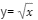are the pixel values of the image data, If x is equal to 1, 4, 9, the value of y is easily calculated as 1, 2, 3,

but if x=4.2, the value of   is not easily calculated.

In this case, you can use the method of calculating the approximate value using the derivative to facilitate the calculation.

< Approximation formulas with derivatives >

f(4+0.2)=f(4)+f'(4)x0.2, (Almost the same as the exact value of 2.049.) So, using the derivative is not completely accurate, but it is simple and allows us to quickly approximate.

Considering this, the invention is based on the idea that the initial data subject to noise addition is composed of the data component (RGB pixel values) + auxiliary variable (pixel values differentiated by time), of which noise can only be added to auxiliary variables.

Specifically, if we assume that predicting the coordinates of an object at a certain time step t after subtracting noise is predicting the coordinates of that object at a certain time, we can express this by comparing the case of adding noise to the existing data itself (position coordinates) to adding noise to the auxiliary variable (velocity = change in position coordinates).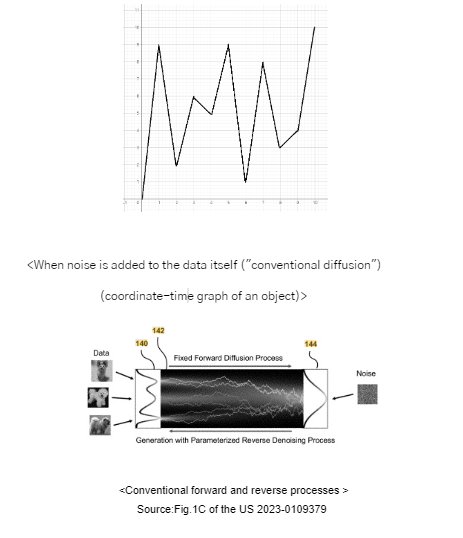As you can see, conventional diffusion method adds noise to the data itself, making it somewhat difficult to predict denoised data at a given time step due to the complexity of the data path over time.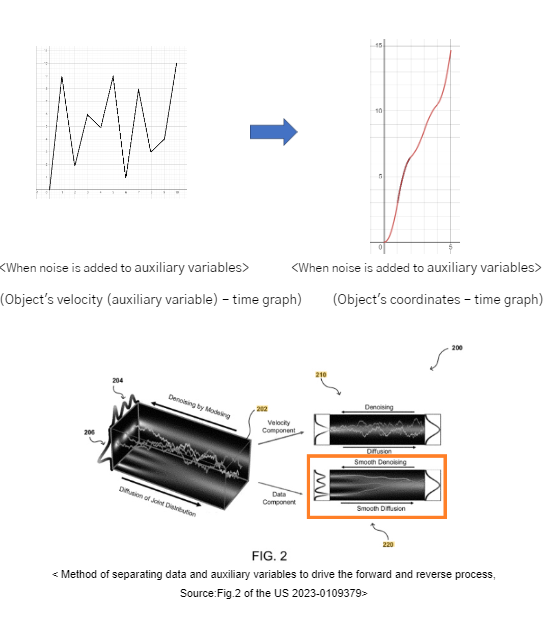On the other hand, if you add noise to an auxiliary variable (difference of the data), the path of the data may be smoother than if you add noise to the data itself, and it may be easier to predict denoised data at a given time step.

This means that by predicting the noise contained in the difference of the data, the method can approximate the computation of predicting the data itself without the noise, which makes the computation simpler than it would otherwise be, allowing for faster sampling rates sustaining the quality of the results.

In this regard, the claims of the invention recite the following.

 A processor, comprising: one or more circuits to cause the processor to perform operations comprising: providing input to a generative neural network;   determining a set of auxiliary values corresponding to a set of data values of the input;   introducing noise values to the set of auxiliary values corresponding to the input to obtain noise data, the one or more noise values being introduced iteratively during a forward diffusion process; removing one or more noise values of the noise values from the noise data to obtain a reconstructed input, the one or more noise values being removed iteratively during a reverse denoising diffusion process; and adjusting network parameters for the score-based generative model based at least on differences between at least the input and the reconstructed input.

Here's how we analyzed the scope of the patent through the currently published claims.

Above all, the patent describes a configuration for (1) determining a set of auxiliary values corresponding to a set of data values of the input, (2) performing a diffusion process (forward diffusion process and reverse denoising diffusion process) on the set of auxiliary data values rather than the input data itself.

First, looking at the configuration of (1) determining a set of auxiliary values corresponding to a set of data values of the input, the claim does not limit the input data and auxiliary data to images, so the configuration is open to multiple meanings.

For example, stable diffusion, which has gotten a lot of attention lately, consists of training to generate a feature map from the input image and adding noise to the feature map, rather than adding noise to the input image itself.

Thus, compared to the claimed representations, it appears that the feature map disclosed in stable diffusion can correspond to the configuration of the set of auxiliary values corresponding to a set of data values of the input, and the training method based on adding noise to the feature map can correspond to the configuration of performing diffusion processes (forward diffusion process and reverse denoising diffusion process) on the auxiliary data value set rather than on the input data itself.

If Nvidia's patent is granted as is, it appears to be a fairly strong patent that could make claims across methods of performing the diffusion process by processing input data into different formats. As such, it will be worth continuing to monitor how the claims of the patent are finalized to avoid infringement issues if it is granted.

So far, we've analyzed the patents filed related to diffusion models and discussed  important sample patents in the image domain. Nowadays, we're seeing a lot of inventions using diffusion models not only in the image domain, but also in the video and 3D domains. So why is the image domain so dominant compared to other domains?

In the next column, we'll share with you the differences between images and other domains, why we haven't seen many patents in other domains compared to the image domain, and what patents have appeared in other domains. Stay tuned for more.

TrendsReportsIP EducationPatentAnalysisSoftware

"Have a question?"